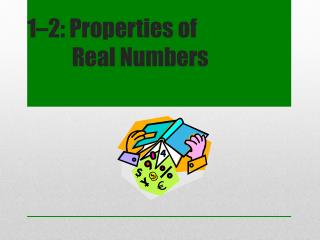# 1 –2: Properties of Real Numbers - PowerPoint PPT PresentationDownload Presentation1 –2: Properties of Real Numbers

1 –2: Properties of Real NumbersDownload Presentation## 1 –2: Properties of Real Numbers

- - - - - - - - - - - - - - - - - - - - - - - - - - - E N D - - - - - - - - - - - - - - - - - - - - - - - - - - -
##### Presentation Transcript

1. 1–2: Properties of Real Numbers

2. {1, 2, 3, 4, 5, …} Counting (Natural) Numbers

3. {0, 1, 2, 3, 4, 5, …} Whole Numbers

4. {…–3, –2, –1, 0, 1, 2, 3 …} Integers

5. All numbers that can be expressed as a/b, where both a and b are integers and b 0. • Includescommon fractions, terminating decimals, repeating decimals, and integers. • They do not include non-repeating decimals, such as . Rational Numbers

6. Irrational Numbers • Those numbers that cannot be expressed as a ratio of two integers • Includes non-terminating, non-repeatingdecimals and special numbers, such as πand

7. All Real Numbers Rational Numbers Irrational Numbers Integers Whole Numbers Counting Numbers

8. All whole numbers are integers. • All integers are whole numbers. • All natural numbers are real numbers. • All irrational numbers are real numbers. Ponder the statements...True or False?

9. Classify each of the following numbers using all the terms that apply: natural (counting), whole, integer, rational, irrational, and real. Classifying Numbers A) B) 3 C) D) –7

10. Closure Property • Commutative Property • Associative Property • Identity Property • Inverse Property • Distributive Property • Properties of Equality Properties of Real Numbers

11. When you combine any two numbers in a set, the answer is part of the set. • For example, when you add or multiply real numbers, the result is also a real number. a + b is a real number a x b is a real number • Learn more Closure Property

12. Commutative means that the order does not make any difference. a + b = b + a a • b = b • a Examples 4 + 5 = 5 + 4 2 • 3 = 3 • 2 • The commutative property does not work for subtraction or division. Commutative Property

13. Associative means that the grouping does not make any difference. (a + b) + c = a + (b + c) (ab) c = a (bc) Examples (1 + 2) + 3 = 1 + (2 + 3) (2 • 3) • 4 = 2 • (3 • 4) • The associative property does not work for subtraction or division. Associative Property

14. Do not change the value! • Additive Identity – When you add zero to any number, the result is the same number a + 0 = a -6 + 0 = -6 • Multiplicative Identity – When you multiply a number by one, the result is the same number a • 1 = a 8 • 1 = 8 Identity Properties

15. Inverse Properties • Undo an operation • Additive Inverse – when you add a number and its opposite, the result is 0 a + (-a) = 0 5 + (-5) = 0 • Multiplicative Inverse – when you multiply a number and its reciprocal, the result is 1

16. Distributive property of multiplication with respect to either addition or subtraction. • a(b + c) = ab + bc • 3(4 - 7) = 3(4) - 3(7) • 3(2x + 4) = 3(2x) + 3(4) = 6x + 12 Distributive property

17. Properties of Equality • Reflexive a = a • Symmetric If a = b, then b = a • Transitive If a = b and b = c, then a = c

18. Real Numbers (mathisfun) • Properties of Real Numbers (regentsprep) • Math Properties (purplemath) • Properties of Equality (hotmath) • Glossary of Properties (dr.math/mathforum) More info…